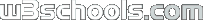Search W3Schools :
XPath Tutorial
XPath HOME
XPath Intro
XPath Nodes
XPath Syntax
XPath Axes
XPath Operators
XPath Examples
XPath Summary

References
XPath Functions

# XPath Operators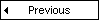An XPath expression returns either a node-set, a string, a Boolean, or a number.

## XPath Operators

Below is a list of the operators that can be used in XPath expressions:

 Operator Description Example Return value | Computes two node-sets //book | //cd Returns a node-set with all book and cd elements + Addition 6 + 4 10 - Subtraction 6 - 4 2 * Multiplication 6 * 4 24 div Division 8 div 4 2 = Equal price=9.80 true if price is 9.80 false if price is 9.90 != Not equal price!=9.80 true if price is 9.90 false if price is 9.80 < Less than price<9.80 true if price is 9.00 false if price is 9.80 <= Less than or equal to price<=9.80 true if price is 9.00 false if price is 9.90 > Greater than price>9.80 true if price is 9.90 false if price is 9.80 >= Greater than or equal to price>=9.80 true if price is 9.90 false if price is 9.70 or or price=9.80 or price=9.70 true if price is 9.80 false if price is 9.50 and and price>9.00 and price<9.90 true if price is 9.80 false if price is 8.50 mod Modulus (division remainder) 5 mod 2 1WAPT is a load, stress and performance testing tool for websites and web-based applications. In contrast to "800-pound gorilla" load testing tools, it is designed to minimize the learning curve and give you an ability to create a heavy load from a regular workstation. WAPT is able to generate up to 3000 simultaneously acting virtual users using standard hardware configuration. Virtual users in each profile are fully customizable. Basic and NTLM authentication methods are supported. Graphs and reports are shown in real-time at different levels of detail, thus helping to manage the testing process. Download the free 30-day trial!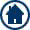HOME or Top of Page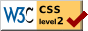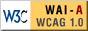Printer Friendly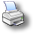W3Schools is for training only. We do not warrant the correctness of its content. The risk from using it lies entirely with the user. While using this site, you agree to have read and accepted our terms of use and privacy policy. Copyright 1999-2009 by Refsnes Data. All Rights Reserved.